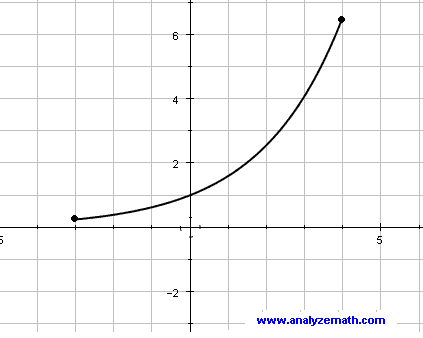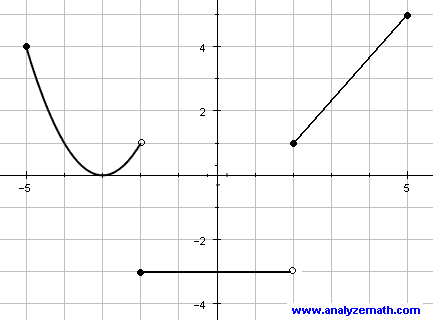# College Algebra Problems With Answers sample 4 : Graphs of Functions

A set of college algebra problems on graphs of functions with answers, are presented. The questions are related to the transformations of graphs, graphs of inverse functions, symmetry of graphs, reading values from graphs, find domain and range and intervals on increase and decrease. The solutions are at the bottom of the page.

## Question 1

The graph of f(x) is shown below. Draw the graph of y = - f(x - 3) - 2Figure 1. Graph of Function f - Question 1

## Question 2

Complete the graph given below so that it is symmetric with respect to the origin.Figure 2. Graph of Question 2

## Question 3

The graph of f(x) is shown below. Sketch the graph of y = f(-x + 1) - 1 (hint: see Graphing by Translation, Scaling and Reflection )Figure 3. Graph of Function f - Question 3

## Question 4

The graph of f(x) is shown below. Sketch the graph of the inverse of f .Figure 4. Graph of Function f - Question 4

## Question 5

The graph of h(x) is shown below.Figure 5. Graph of Function f - Question 5
a) evaluate: h(-2) + h(2) =
b) What is the domain of h?
c) What is the range of h?
d) Find the interval(s) over which h is increasing.
e) Find the interval(s) over which h is decreasing.
f) Find the interval(s) over which h is constant.

## Question 6

The graph of f(x) is shown below. Sketch the graph of f(2x) .Figure 6. Graph of Function f - Question 6

## Question 7

The graph of f -1(x) is shown below.
Evaluate the following: f(0) , f(2)

## Solution to Question 1

Select points whose coordinates are easy to determine on the given graph (see graph in black below) and then transform them as follows:
1 - shift right 3 units : f(x - 3)
2- reflect on the x-axis : - f(x - 3)
3 - shift down 2 units : - f(x - 3) - 2
4 - connect the transformed points to sketch the transformed graph shown in red below.

## Solution to Question 2

A graph is symmetric with respect to the origin if for each point (a,b) on the graph there exists a point (-a,-b) on the same graph.
We select points (a,b) on the given graph and then transform them into (-a,-b) to obtain more points. When put together the graph is symmetric with respect to the origin.(see the whole graph black and red below).

## Solution to Question 3

Select points on the given graph and then transform them as follows:
1 - reflect on the y - axis : f(-x)
2- shift right one unit: f(- x + 1) = f(-(x - 1))
3 - shift down 1 unit : f(- x + 1) - 1
4 - connect the transformed points to sketch the transformed graph shown in red below.

## Solution to Question 4

We first determine points (a , b) on the graph of the given function and then use the definition of the inverse to determine points (b , a) on the graph of the inverse. Or use the line y = x to reflect points (a , b) into (b , a).Figure 11. Graph of Solution to Question 4

## Solution to Question 5

h(-2) + h(2) = -3 + 1 = -2
Domain: [-5 , 5]
Range: {-3} U [0 , 5]
increase: [-3 , 2) , [2 , 5]
decrease: [-5 , -3]
constant: [-2 , 2)

## Solution to Question 6

Function f has two x-intercepts: x = 2 and x = -2 . f(2x) will also have x-intercepts such that 2x = 2 which gives x = 1 and 2x = -2 which gives x = -1. Hence f(2x) will have x intercepts at x = 1 and x = -1. The y-intercept is at y = 2 since f(0) = 2 and f(2*0) = f(0) = 2.Figure 12. Graph of Solution to Question 6

## Solution to Question 7

since f -1 (1) = 0 , f(0) = 1 , and since f -1 (0) = 2 , f(2) = 0